# Texas Go Math Grade 4 Lesson 13.3 Answer Key Parallel Lines and Perpendicular Lines

Refer to our Texas Go Math Grade 4 Answer Key Pdf to score good marks in the exams. Test yourself by practicing the problems from Texas Go Math Grade 4 Lesson 13.3 Answer Key Parallel Lines and Perpendicular Lines.

## Texas Go Math Grade 4 Lesson 13.3 Answer Key Parallel Lines and Perpendicular Lines

Essential Question

How can you identify and draw parallel lines and perpendicular lines?
Parallel lines are lines in a plane that always have the same distance apart. Parallel lines never intersect each other. Perpendicular lines are lines that intersect at a right (90 degrees) angle.

Explanation:
are lines that meet at right angles.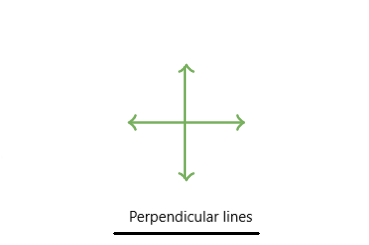Parallel lines are always the same distance apart from each other — no matter how far they are extended, they will never meet.Unlock the Problem

You can find models of lines in the world around you. For example, two streets that cross each other model intersecting lines. Metal rails on a train track that never cross model parallel lines.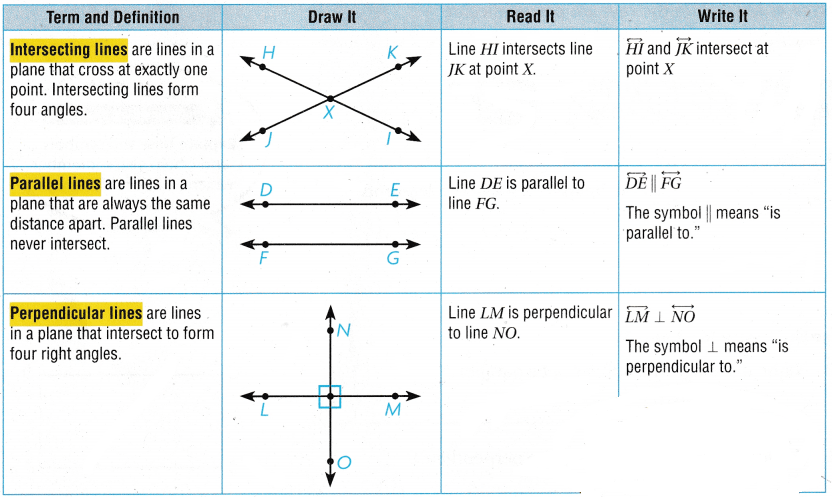Math Talk

Mathematical Processes
Can two rays be parallel? Explain.
Yes, two rays can be parallel.

Explanation:
Two rays can be parallel because a ray is a part of a line.

Try This! Tell how the streets appear to be related. Write perpendicular, parallel, or intersecting.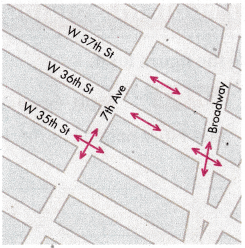• W 36 th St and Broadway _____________________
• W 35 th St and 7 th Ave _____________________
• W 37 th St and W 36th St _____________________

W 36 th St and Broadway intersect at each other.
W 35 th St and 7 th Ave is perpendicular to each other.
W 37 th St and W 36th St is parallel to each other.

Activity Draw and label $$\overrightarrow{Y X} \perp \overrightarrow{Y Z}$$. intersecting at point Y.

Materials

• straightedge

STEP 1: Draw and label $$\overrightarrow{Y X}$$.

STEP 2: Then draw and label $$\overrightarrow{Y Z}$$.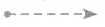STEP 3: Make sure $$\overrightarrow{Y X}$$ and $$\overrightarrow{Y Z}$$ intersect at point Y.

STEP 4: Make sure the rays are perpendicular.

How can you check if two rays are perpendicular?

Share and Show

Question 1.
Draw and label $$\overline{Q R} \| \overline{S T}$$.
Think: Parallel lines never intersect. Parallel line segments are parts of parallel lines.Math Talk

Mathematical Processes
Explain how the symbols ⊥ and || help you remember which relationships they describe.

Use the figure for 2 and 3.Question 2.
Name two sides that appear to be parallel.
BF and CD
BC and DF

Explanation:

Question 3.
Name two sides that appear to be perpendicular.
CB is perpendicular to BF
DF is perpendicular to BF

Problem Solving

Use the figure for 4.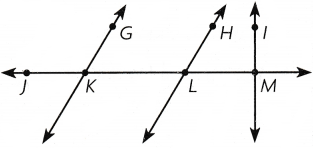Question 4.
H.O.T. Multi step What’s the Error? Dan says that $$\overleftrightarrow{H L}$$ is parallel to $$\overleftrightarrow{I M}$$. Is Dan correct? Explain.
No, the two lines intersect.

Explanation:
There is an error in what Dan said. Here the $$\overleftrightarrow{H L}$$ is not parallel to $$\overleftrightarrow{I M}$$. As $$\overleftrightarrow{I M}$$ is a perpendicular line which has 90° and $$\overleftrightarrow{H L}$$ has an obtuse angle that is more than 90°.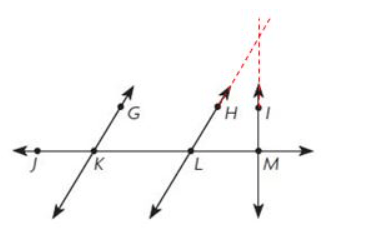Problem Solving

Use the house plan at the right for 5-7.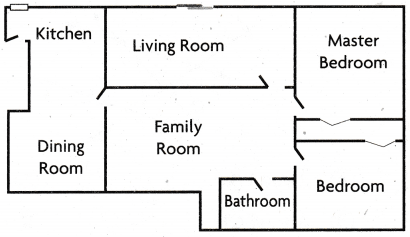Question 5.
What geometric term describes a corner of the living room?
Vertex

Explanation:
The answer is a vertex. Corners of any shape are vertices.

Question 6.
Use Diagrams Name three parts of the plan that show line segments.
A line segment is a straight line which links two points without extending beyond them. In other words, any line that is a finite line is a line segment.

Explanation:Question 7.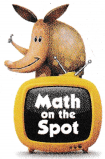H.O.T. Name a pair of line segments that appear to be parallel.
Living room and Dining room.

Explanation:Use the map at the right for 8-10.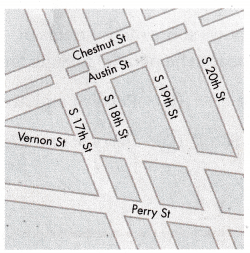Question 8.
Name a Street that appears to be parallel to S 17th Street.
S 18th Street.

Explanation:
A Street that appears to be parallel to S 17th Street is S 18th Street.

Question 9.
Name a Street that appears to be parallel to Vernon Street.
Perry street

Explanation:
A Street that appears to be parallel to Vernon Street is Perry Street.

Question 10.
Name a Street that appears to be perpendicular to s 19th Street.
Austin St

Explanation:
A Street that appears to be perpendicular to s 19th Street is Austin St.

Question 11.
Which pair of lines appear to he parallel?(A) $$\overleftrightarrow{A B}$$ and $$\overleftrightarrow{Q X}$$
(B) $$\overleftrightarrow{A B}$$ and $$\overleftrightarrow{C Y}$$
(C) $$\overleftrightarrow{C Y}$$ and $$\overleftrightarrow{Z R}$$
(D) $$\overleftrightarrow{Z R}$$ and $$\overleftrightarrow{Q X}$$
Option C

Explanation:
The $$\overleftrightarrow{C Y}$$ and $$\overleftrightarrow{Z R}$$ pair of lines appear to be parallel.

Question 12.
Which figure appears to show 1? I
(A)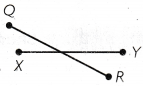(B)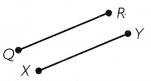(C)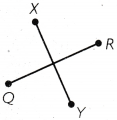(D)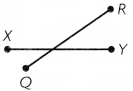Option B

Explanation:
QR and XY are parallel to each other.

Question 13.
Multi-Step The map below shows some of the streets in Matt’s town. Which streets appear to be parallel to State Street?
(A) Chestnut St and Austin St
(B) 17th St, 18th St, and 19th St
(C) Perry St and Austin St
(D) Perry St and 17th St
Chestnut St and Austin St

Explanation:
The streets Chestnut St and Austin St appear to be parallel to State Street.

‘TEXAS Test Prep

Question 14.
Which best describes perpendicular lines?
(A) They never meet.
(B) They form four right angles.
(C) They form one acute angle.
(D) They form one obtuse angle.
They form four right angles.

### Texas Go Math Grade 4 Lesson 13.2 Homework and Practice Answer Key

Use the figure for 1-3.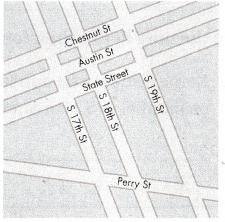Question 1.
Name two lines that appear to be parallel.
Chestnut St and Austin St.

Question 2.
Name two lines that appear to be perpendicular.
State Street and S 17th St.

Question 3.
Name two lines that intersect but do not appear to be perpendicular.
S 17th St and Perry St.

Question 4.
Connie says that Main St. appears to be parallel to Elm St. Is Connie correct? Explain.

Question 5.
Daniel says that Main St. and 3rd Street appear to be perpendicular. Is Daniel correct? Explain.

Lesson Check

Question 6.
Which pair of lines appear to be perpendicular?(A) $$\overleftrightarrow{J K}$$ and $$\overleftrightarrow{L M}$$
(B) $$\overleftrightarrow{L M}$$ and $$\overleftrightarrow{G H}$$
(C) $$\overleftrightarrow{L M}$$ and $$\overleftrightarrow{T R}$$
(D) $$\overleftrightarrow{T R}$$ and $$\overleftrightarrow{G H}$$
$$\overleftrightarrow{L M}$$ and $$\overleftrightarrow{T R}$$

Explanation:
The pair of lines appear to be perpendicular is $$\overleftrightarrow{L M}$$ and $$\overleftrightarrow{T R}$$.

Question 7.
Which figure appears to show parallel lines?
(A)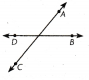(B)(C)(D)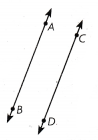Option D

Explanation:
Option D in the given figure appears which shows parallel lines.

Question 8.
Which best describes parallel lines?
(A) They never meet.
(B) They intersect at one point.
(C) They form one acute angle.
(D) They form one obtuse angle.
They never meet

Explanation:
Parallel lines will never meet each other.

Question 9.
Which does NOT describe intersecting lines?
(A) They cross at exactly one point.
(B) They form four angles.
(C) They are the same distance apart.
(D) They may form a right angle.
They are the same distance apart.

Explanation:
The intersecting lines will not have the same distance.

Question 10.
Multi-Step The map shows some of the streets in Diana’s town. Which streets appear to be perpendicular to S 19th St?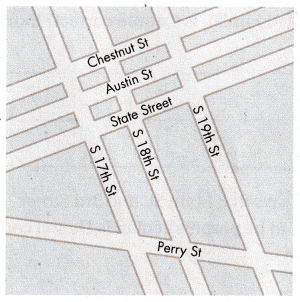(A) Austin St and State St
(B) Chestnut St and Perry St
(C) State St and S 18th St
(D) S 17th St and S 18th St
Austin St and State St

Explanation:
The streets Austin St and State St appear to be perpendicular to S 19th St.

Question 11.
Multi-Step Which streets appear to be parallel to S 17th St?
(A) State St and Chestnut St
(B) S 18th St and S 19 St
(C) Perry St and Austin St
(D) S 19 St and Perry St